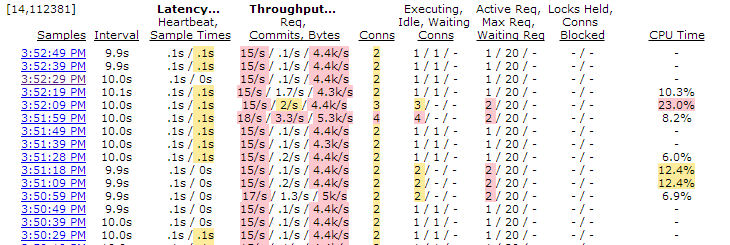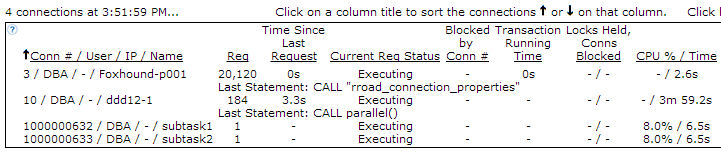# Intra-Procedure Parallelism

This is an experimental re-post of the article first published over here. For the most part the differences between the Google Blogger editor and the editor used on this site are minor… annoying, but minor.

The big roadblock so far is line numbering in the SQL code, something this article depends on… line numbering is possible but not easy.

Question: How can I make a SQL Anywhere stored procedure run faster?

Answer: One way is to make use of the excess CPU capacity found on many computers by spreading the work across two or more CPUs… not by relying on intra-query parallelism which works on individual SQL statements, but by moving blocks of code out of a single stored procedure into separate events so they run in parallel on separate internal connections.

The title calls this technique “Intra-Procedure Parallelism” but it’s really an example of “inter-query parallelism” which is descibed in the Help as “executing different requests simultaneously on separate CPUs”… in this case, the original code that executed as a single request (one procedure call) is changed into separate requests (event invocations) that execute simultaneously. Inter-query parallelism has been around forever in SQL Anywhere, and this article shows how it can be exploited to improve the performance of a single user connection.

Here’s an example, a procedure called “serial” that performs two time-consuming steps one after the other:

1. CREATE TABLE t (
2.    result_number   INTEGER NOT NULL PRIMARY KEY,
3.    result          BIGINT NOT NULL );
4.
5. CREATE PROCEDURE serial()
6. BEGIN
7.
8. DECLARE @start        TIMESTAMP;
9. DECLARE @start_step_1 TIMESTAMP;
10. DECLARE @start_step_2 TIMESTAMP;
11.
12. — Step 1.
13.
14. SET @start        = CURRENT TIMESTAMP;
15. SET @start_step_1 = CURRENT TIMESTAMP;
16.
17. INSERT t
18. ON EXISTING UPDATE
19. SELECT 1,
20.        SUM ( CAST ( a.row_num AS BIGINT ) )
21.   FROM RowGenerator AS a
22.        CROSS JOIN RowGenerator AS b
23.        CROSS JOIN RowGenerator AS c;
24. COMMIT;
25.
26. MESSAGE STRING ( CAST ( DATEDIFF ( MILLISECOND, @start_step_1, CURRENT TIMESTAMP )
27.                         AS DECIMAL ( 11, 2 ) ) / 1000.0,
28.                  ‘ seconds to perform step 1’ ) TO CONSOLE;
29.
30. — Step 2.
31.
32. SET @start_step_2 = CURRENT TIMESTAMP;
33.
34. INSERT t
35. ON EXISTING UPDATE
36. SELECT 2,
37.        SUM ( CAST ( a.row_num AS BIGINT ) )
38.   FROM RowGenerator AS a
39.        CROSS JOIN RowGenerator AS b
40.        CROSS JOIN RowGenerator AS c
41.        CROSS JOIN RowGenerator AS d
42. WHERE d.row_num <= 2;
43. COMMIT;
44.
45. MESSAGE STRING ( CAST ( DATEDIFF ( MILLISECOND, @start_step_2, CURRENT TIMESTAMP )
46.                         AS DECIMAL ( 11, 2 ) ) / 1000.0,
47.                  ‘ seconds to perform step 2’ ) TO CONSOLE;
48.
49. MESSAGE STRING ( CAST ( DATEDIFF ( MILLISECOND, @start, CURRENT TIMESTAMP )
50.                         AS DECIMAL ( 11, 2 ) ) / 1000.0,
51.                  ‘ seconds to perform both steps’ ) TO CONSOLE;
52.
53. END;
54.
55. CALL serial();
56.
57. 10.1680000 seconds to perform step 1
58. 19.9700000 seconds to perform step 2
59. 30.1440000 seconds to perform both steps

Both steps ran on the same CPU, ignoring all other processors that might be sitting idle, and together they took a total of 30 seconds to run.

Here’s the alternative, a procedure called “parallel” that uses the TRIGGER EVENT statement to launch two events, “subtask1” and “subtasks2”:

• The code on lines 14 through 21 initializes subtask_in_progress with a “not yet started” row for each subtask; the CONNECTION_PROPERTY ( ‘Number’ ) function is used to fill in the task_connection_number, and subtask_connection_number is set to zero.

• The TRIGGER EVENT statements on lines 25 and 26 take almost zero time to execute because they are “fire and forget” operations, not “call and return”.

• The code on lines 28 through 37 waits for all the subtask events to get underway, then the code on lines 39 through 57 waits until they are all complete.

• It is important to note that the subtask events (shown later) fill in subtask_in_progress.subtask_connection_number values, but only the caller sets those values back to zero (lines 48 through 55) when they no longer match actual connections. Stuff happens; the subtask events can terminate abnormally, so they can’t be relied upon to tell the caller when they’re done.
3.    subtask_number              INTEGER NOT NULL, — 1, 2
6.
7. CREATE PROCEDURE parallel()
8. BEGIN
9.
10. DECLARE @start TIMESTAMP;
11.
12. SET @start = CURRENT TIMESTAMP;
13.
14. — Initialize subtasks as “not yet started”.
15.
17. WHERE task_connection_number = CONNECTION_PROPERTY ( ‘Number’ );
18.
19. INSERT subtask_in_progress VALUES ( CONNECTION_PROPERTY ( ‘Number’ ), 1, 0 );
20. INSERT subtask_in_progress VALUES ( CONNECTION_PROPERTY ( ‘Number’ ), 2, 0 );
21. COMMIT;
22.
24.
27.
28. — Wait for all the subtasks to start.
29.
30. WHILE EXISTS ( SELECT *
32.                 WHERE task_connection_number = CONNECTION_PROPERTY ( ‘Number’ )
33.                   AND subtask_connection_number = 0 ) LOOP
34.
35.    WAITFOR DELAY ’00:00:00.5′;
36.
37. END LOOP;
38.
39. — Wait for all the subtasks to finish.
40.
41. WHILE EXISTS ( SELECT *
43.                 WHERE task_connection_number = CONNECTION_PROPERTY ( ‘Number’ )
44.                   AND subtask_connection_number <> 0 ) LOOP
45.
46.    WAITFOR DELAY ’00:00:00.5′;
47.
48.    — Update any of the subtasks that have finished.
49.
52.     WHERE task_connection_number = CONNECTION_PROPERTY ( ‘Number’ )
54.       AND CONNECTION_PROPERTY ( ‘Number’, subtask_connection_number ) IS NULL;
55.    COMMIT;
56.
57. END LOOP;
58.
59. MESSAGE STRING ( CAST ( DATEDIFF ( MILLISECOND, @start, CURRENT TIMESTAMP )
60.                         AS DECIMAL ( 11, 2 ) ) / 1000.0,
61.                  ‘ seconds to perform both tasks’ ) TO CONSOLE;
62.
63. END;
64.
65. CALL parallel();
66.
67. 11.7720000 seconds to perform subtask1
68. 21.5570000 seconds to perform subtask2
69. 21.8280000 seconds to perform both tasks

Each subtask event does take longer than corresponding step in the serial solution, but the whole process is a lot faster: 22 seconds instead of 30.

Here’s the code for the two subtask events:

• The CREATE EVENT statements on lines 1 and 33 show how to create “untyped” or “user-defined” events; i.e., events that have neither TYPE nor SCHEDULE clauses and can thus be fired only by TRIGGER EVENT statements.

• Lines 8 through 14 and 40 through 46 show how the rows in subtask_in_progress are updated; EVENT_PARAMETER ( ‘ConnectionID’ ) provides the caller’s connection number, and CONNECTION_PROPERTY ( ‘Number’ ) gives the event’s connection number (developers from The Old School might prefer to use @@SPID).

• Lines 16 through 25 is the code from step 1 in the serial solution, and 48 through 59 is the code from step 2.
2. HANDLER BEGIN
3. DECLARE @start TIMESTAMP;
4. SET @start = CURRENT TIMESTAMP;
5. — Mark subtask1 as “started”.
7.    SET subtask_connection_number = CONNECTION_PROPERTY ( ‘Number’ )
8. WHERE task_connection_number = EVENT_PARAMETER ( ‘ConnectionID’ )
10. COMMIT;
11. — Do step 1.
12. INSERT t
13. ON EXISTING UPDATE
14. SELECT 1,
15.        SUM ( CAST ( a.row_num AS BIGINT ) )
16.   FROM RowGenerator AS a
17.        CROSS JOIN RowGenerator AS b
18.        CROSS JOIN RowGenerator AS c;
19. COMMIT;
20. MESSAGE STRING ( CAST ( DATEDIFF ( MILLISECOND, @start, CURRENT TIMESTAMP )
21.                         AS DECIMAL ( 11, 2 ) ) / 1000.0,
22.                  ‘ seconds to perform subtask1’ ) TO CONSOLE;
23. END;
25. HANDLER BEGIN
26. DECLARE @start TIMESTAMP;
27. SET @start = CURRENT TIMESTAMP;
28. — Mark subtask2 as “started”.
30.    SET subtask_connection_number = CONNECTION_PROPERTY ( ‘Number’ )
31. WHERE task_connection_number = EVENT_PARAMETER ( ‘ConnectionID’ )
33. COMMIT;
34. — Do step 2.
35. INSERT t
36. ON EXISTING UPDATE
37. SELECT 2,
38.        SUM ( CAST ( a.row_num AS BIGINT ) )
39.   FROM RowGenerator AS a
40.        CROSS JOIN RowGenerator AS b
41.        CROSS JOIN RowGenerator AS c
42.        CROSS JOIN RowGenerator AS d
43. WHERE d.row_num <= 2;
44. COMMIT;
45. MESSAGE STRING ( CAST ( DATEDIFF ( MILLISECOND, @start, CURRENT TIMESTAMP )
46.                         AS DECIMAL ( 11, 2 ) ) / 1000.0,
47.                  ‘ seconds to perform subtask2’ ) TO CONSOLE;
48. END;

The CPU usage for the serial and parallel solutions shows how using 2 processors instead of just one can reduce the overall elapsed time from 30 seconds to 22:

Serial CPU Usage

Parallel CPU UsageHere’s another look at the two tests using Foxhound, with the serial test appearing below (earlier in time) than the parallel test:

Serial and Parallel TestsHere’s what the connections look like during the serial and parallel tests:

Serial Connection

Parallel ConnectionsIn this example it wasn’t actually necessary to create two subtask events, one would have sufficed: the subtask1 event could have handled step 1 and the calling procedure could have performed step 2 and then checked to make sure subtask1 was finished.

As coded, there are three connections in use, two doing actual work (subtask1 and subtask1) and one leaning on a shovel… 🙂

/